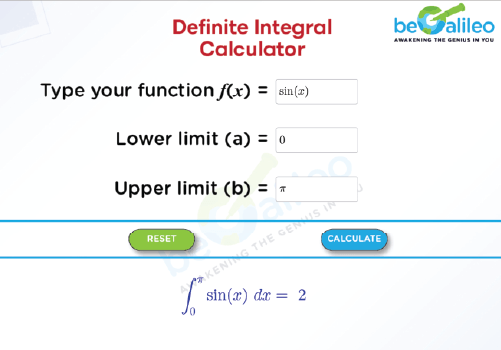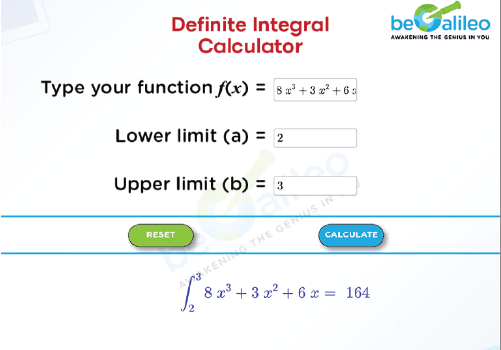>### Master Math with 1:1 Online Classes. Learn from Experts!# Definite integral Calculator

A definite integral calculator is a tool or software program designed to compute definite integrals of mathematical functions. Definite integrals are used in calculus to find the area under a curve between two points on the x-axis (limits of integration). This calculation can be complex, especially for functions with intricate formulas, so definite integral calculators are valuable for performing these calculations quickly and accurately.

## How to use the Definite Integral Calculator?

1. Function Entry :
You provide the mathematical function that you wish to integrate. This function represents the curve for which you want to find the area under or other quantities related to integration.
2. Limits Specification :
In order to determine the range across which you want to get the definite integral, you must provide the lower and upper limits of integration (denoted as ‘a’ and ‘b’) respectively.
3. Calculation :
The calculator then applies numerical methods or algorithms to approximate the definite integral of the given function over the specified interval. It may use techniques such as the trapezoidal rule, Simpson's rule, or more advanced numerical integration methods.
4. Result Display :
The calculator provides you with the numerical result of the definite integral, usually rounded to a certain number of decimal places. The result will be displayed below the calculator.
5. Reset Option :
If needed, you can reset everything to their default settings by clicking the "Reset" button.

These steps will help you efficiently use the Definite Integral Calculator to compute definite integrals.

## How to find Definite integral?

1. Identify the Function and Interval :
Let us say you have a function f(x) and want to find its definite integral from a to b. The notation for this is :
\int_{\text{a}}^{\text{b}} \text{f(x) dx}
2. Find the Antiderivative (Indefinite Integral) :
To calculate the definite integral, you first need to find the antiderivative (indefinite integral) of the function f(x). The antiderivative is a function F(x) such that F'(x)=f(x).
3. Apply the Fundamental Theorem of Calculus :
According to the Fundamental Theorem of Calculus, the definite integral of f(x)from a to b can be calculated as follows :
\int_{\text{a}}^{\text{b}} \text{f(x) dx = F(b)-F(a)}; Where F(x) is the antiderivative of f(x).
4. Evaluate the Integral :
Substitute the upper limit (b) and lower limit (a) of integration into the antiderivative F(x):
\int_{\text{a}}^{\text{b}} \text{f(x) dx = F(b)-F(a)}
5. Simplify and Calculate :
Calculate F(b) and F(a) by substituting b and a into the antiderivative F(x). Subtract F(a) from F(b) to obtain the numerical value of the definite integral.
6. Finalize the Result :
The result is the numerical value of the definite integral of f(x) from a to b.

### Example :

Find the definite integral of the function f(x)=2x from x = 1 to x = 4.
Solution :
We want to calculate the definite integral of f(x)=2x over the interval [1, 4].
We can write this as, \int_{1}^{4} \text{2x dx}
\int_{1}^{4} \text{2x dx}
= 2\int_{1}^{4} \text{x dx}
=2\left[\frac{\text{x}^2}{2}\right]_1^4
=\left[\text{x}^2\right]_1^4
=\ 4^2 -1^2
= 16-1
= 15
Therefore ,\int_1^4 \text{2x dx}=15

## Solved examples

### Q1. Find the definite integral of the function f(x)=sin(x) dx from x = 0 to x=\pi.

Solution :
\int_{0}^{\pi}\text{sin(x) dx = [-cos(x)]}_{0}^{\pi}
= -(cos(\pi)-cos(0))
= -(-1-1)
= -(-2)
= 2
Therefore ,\int_{0}^{\pi}\text{sin(x) dx = 2}### Q2. Find the definite integral of the function f(x) =\text{8x}^3+\text{3x}^2+6x dx from x = 2 to x = 3.

Solution :
\int_{2}^{3}(8x^3+3x^2+6x)\ dx=\int_{2}^{3} 8x^3 \ dx+ \int_{2}^{3} 3x^2 \ dx +\int_{2}^{3} 6x \ dx
=8\int_{2}^{3} x^3 \ dx+ 3\int_{2}^{3} x^2 \ dx +6\int_{2}^{3} x \ dx
=8\left[\frac{\text{x}^4}{4}\right]_2^3 + 3\left[\frac{\text{x}^3}{3}\right]_2^3 +6\left[\frac{\text{x}^2}{2}\right]_2^3
=2\left[\text{x}^4\right]_2^3 + \left[\text{x}^3\right]_2^3 +3\left[\text{x}^2\right]_2^3
=2\left[3^4 - 2^4\right] + 2\left[3^3 - 2^3\right] +3\left[3^2 - 2^2\right]
=2(65)+19+3(5)
=130+19+15
=164
Therefore ,\int_{2}^{3}(8x^2+3x^2+6x) dx=164